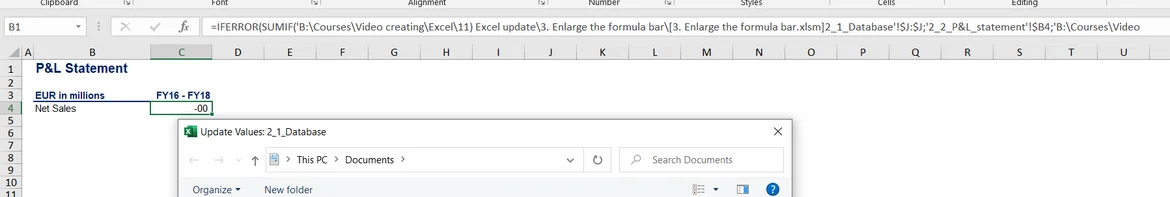Super learner

15 Aug 2023

Posted on:

06 Aug 2023

1

# Resolved:About the last part of the lecture video

I have a problem performing the last part when the link to the external source needs to be replaced.

In the beginning, we have a formula:
=IFERROR(SUMIF('B:\Courses\Video creating\Excel\11) Excel update\3. Enlarge the formula bar\[3. Enlarge the formula bar.xlsm]2_1_Database'!\$J:\$J;'2_2_P&L_statement'!\$B4;'B:\Courses\Video creating\Excel\11) Excel update\3. Enlarge the formula bar\[3. Enlarge the formula bar.xlsm]2_1_Database'!F:F)/1000000;0)+IFERROR(SUMIF('B:\Courses\Video creating\Excel\11) Excel update\3. Enlarge the formula bar\[3. Enlarge the formula bar.xlsm]2_1_Database'!\$J:\$J;'2_2_P&L_statement'!\$B4;'B:\Courses\Video creating\Excel\11) Excel update\3. Enlarge the formula bar\[3. Enlarge the formula bar.xlsm]2_1_Database'!G:G)/1000000;0)+IFERROR(SUMIF('B:\Courses\Video creating\Excel\11) Excel update\3. Enlarge the formula bar\[3. Enlarge the formula bar.xlsm]2_1_Database'!\$J:\$J;'2_2_P&L_statement'!\$B4...

When I am replacing [3. Enlarge the formula bar.xlsm] I get a pop-up window Update Values: 2_1_Database

I do not see the message that replacement has been performed but see that formula has changed to:

=IFERROR(SUMIF('B:\Courses\Video creating\Excel\11) Excel update\3. Enlarge the formula bar\[2_1_Database]2_1_Database'!\$J:\$J;'2_2_P&L_statement'!\$B4;'B:\Courses\Video creating\Excel\11) Excel update\3. Enlarge the formula bar\[2_1_Database]2_1_Database'!F:F)/1000000;0)+IFERROR(SUMIF('B:\Courses\Video creating\Excel\11) Excel update\3. Enlarge the formula bar...

So it seems that the replacement of [3. Enlarge the formula bar.xlsm] has been performed but I constantly obtain pop-up window Update Values: 2_1_Database

What can be the reason and how to fix it? Thank you!Instructor
Posted on:

15 Aug 2023

0

Hi Oleksii,

I apologize for the late reply.

Also, the example I've provided is far from optimal because the name is too long. Having said that, you need to remove the following from all parts of your formula:

B:\Courses\Video creating\Excel\11) Excel update\3. Enlarge the formula bar\[2_1_Database]2_1_Database'!\$J:\$J;'2_2_P&L_statement'!\$B4;'B:\Courses\Video creating\Excel\11) Excel update\3. Enlarge the formula bar\[2_1_Database]

Best,

Ned